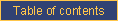homefeaturesexamplesDOCUMENTATIONblogpricingcontact ustechnical documentationCATI documentationcookbookrobotRandomly selecting a subset of selected answer categories

Problem

You want to select a random combination of answers provided to a multiple-answer question.

Solution

Use a CALCUL question with an expression like the following:

RANDOMSELECTION = random_subset(\$MULTIQ,2)

where

• "RANDOMSELECTION" is the name of the multiple-selection question (with a MAX= parameter greater than 1) where the random selection will be stored;
• "MULTIQ" is the name of the multiple-selection question which needs to be subsetted;
• "2" can be any positive integer and corresponds to the number of answers to select.

Discussion

Sometimes, it is necessary to select a random subset of answers provided to a multiple-selection question. An example would be to randomly select two magazines among a list of magazines selected by the respondent. The special function random_subset is used for this purpose.

This function selects a random subset of the answers provided and returns the result as a value that can be used on another multiple-selection question. If the respondent originally offered fewer answers than required in the random subset, all of their answers will be included in the "random subset".

The result of this function can be used in display conditions as if it had been produced in response to a multiple-selection question.

It is possible to randomly select a single answer as well (e.g., RANDOMSELECTION = random_subset(\$MULTIQ,1)); such a selection would be stored in a single-answer question, not a multiple-selection question.e-mail technical support!